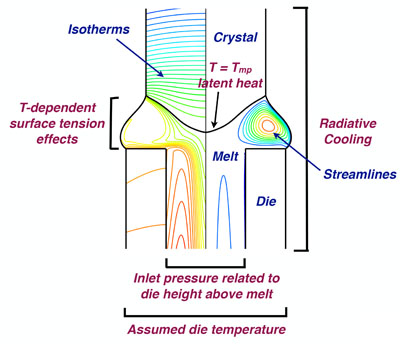Cats2D Multiphysics > Overview > Control file

## Cats2D control file format

Cats2D requires a text input file named <jobtitle>ctrl.txt (default is flowctrl.txt) to define a physical problem to be solved. Simple commands are written to select a coordinate system, to define governing equations for each material, to apply boundary conditions to boundaries, and to define flags that control various aspects of code behavior. Commands and comments can be given in any order, and are written in a syntax that is easy to understand. The lid-driven cavity, for example, requires only two commands:

```flow(on)
bc(u-dirichlet(3,(3,0,0,0),(1,1.0)))```

Here is a more complicated example, the EFG (edge-defined film-fed growth) problem:```geometry(axisymmetric)reference-frame(translational,2)
multiphase(3)material(1,3,3,4,5,liquid)material(2,2,1,2,solid)material(3,1,6,graphite)
% %%%%%%%%%%  ENERGY  %%%%%%%%%%%
energy(on)
% growth interfacebc(latent-heat(1,(0)))
% die bottom and inlet temperaturebc(t-dirichlet(9,(3,1,1,0)))bc(t-dirichlet(4,(3,1,1,0)))
% furnace to melt, crystal side, die, crystal endbc(t-robin(2,(0)))bc(t-robin(7,(0)))bc(heat-flux-user(8,(2,17,5)))bc(heat-flux-user(10,(2,17,5)))
% %%%%%%%%%%  FLOW  %%%%%%%%%%%
flow(on,1)
% meniscusbc(capillary-traction(2,(2,0,0)))
% density change at interfacebc(continuity(1,(2,1,1)))
% die inletbc(outflow(4,(1,0)))
% %%%%%%%%%%  MESH  %%%%%%%%%%%
mesh(on)
% growth interfacebc(isotherm(1,(0)))
% meniscus bc(kinematic(2,(2,0,0)))
% growth angle condition computes y-position of trijunctionbc(angle(2,(3,1,2,0)))
% side of crystalbc(slope(8,(1,0)))
```